Mathematics and Computer Science
Volume 2, Issue 1, January 2017, Pages: 1-5

Investigation of the Numerical Solution of Integral Equation with Kernels Involving Logarithmic Functions

Department of Mathematics, University of Hail, Hail, Saudi Arabia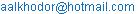Azhari Ahmad Abdalla. Investigation of the Numerical Solution of Integral Equation with Kernels Involving Logarithmic Functions. Mathematics and Computer Science. Vol. 2, No. 1, 2017, pp. 1-5. doi: 10.11648/j.mcs.20170201.11

Received: November 21, 2016; Accepted: November 30, 2016; Published: January 3, 2017

Abstract: The main aim of this paper is to investigate the numerical solution of first kind integral equation of logarithmic kernel when using spectral method. Our approach consists of limiting the boundary to the unit interval and specify a logarithmic kernel. The behavior of the solution on the unit interval was analyzed and the advantages and disadvantages of this approach was shown.

Keywords: Integral Equation, Spectral Method, Logarithmic Kernel

Contents

1. Introduction

Many problems in engineering and science, can be modeled with partial differential equations. If these problems involve some well defined region, Ω, with known conditions on the boundary Γ, then the equations can be transformed into a Fredholm integral equation of the first or second kind, . The integral equation of the first kind with logarithmic kernel ( "Symm's integral equation") is one of the classical integral equations of potential theory. It arises, e.g., from a single layer representation for the solution of the problem [29,4,18,5,6,7], and it has also well-known applications in conformal mappings [8, 10].

In this paper we will consider equations of the first kind: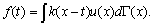g(t)               (1)

where f represents some known data at the point t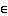Γ and K is a distribution of source (or sink) terms over the boundary . We seek to find the density of this distribution, u.

The integral equation (1) is inherently ill-posed. That is, it can be shown that a small perturbation on f can give rise to an arbitrarily large perturbation in u. To substantiate this point, consider the singular integral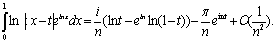(2)

For large n, infinitesimal changes for the integral (right hand side) correspond to finite changes in the integrand. The problem is compounded if instead we consider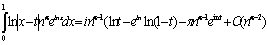(3)

For 0 < α < 1 and n large, then infinitely small changes for the integral correspond to infinitely large changes in the integrand. Even though (2) is within a constant multiple of (3), as n becomes large we can see that small errors in f may lead to finite or infinite errors in u.

For this reason, numerical methods for solving such equations are often ill-fated [16, 18]. The simple illustration here shows this, is often manifested in high frequency terms for the unknown.

In an attempt to compare this method with the analytical solution of (1), we limit the boundary to the unit interval and specify a logarithmic kernel. In addition, we impose the condition that, u, has zero derivative at the endpoints. The kernel and condition on, u, were chosen since these characteristics are present in most problems which exhibit axial symmetry.

We implement here spectral methods to solve Symm’s integral equation on the unit circle [5,8]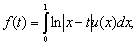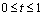(4)

In the specific example used here we haveThe exact solution is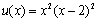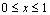(5)

using a spectral approach where the unknown is represented as a finite sum of orthogonal functions . That is,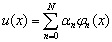0 x 1,                 (6)

where {ϕn} is the orthogonal basis of some inner product space, in which, u, is a member, and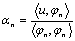n =0,1,………              (7)

are the Euler equations that define the coefficients of the expansion. The method calculates these coefficients and the resulting approximation is thus furnished. There are a few advantages and disadvantages with this approach:

the approximation is valid for the entire domain as no discretization is employed.

the properties of the solution are derived from those of the basis functions { ϕn }. For orthogonal polynomials, and in particular trigonometric polynomials, this would mean that the solution is everywhere analytic. This may not be expedient if one knows that the solution should exhibit some non-analytic behavior. Usually, this property is desirable and non-analytic functions can be catered for by expanding in a weighted series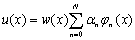(8)

In this case the basis, {ϕn}, would be orthogonal with respect to the weight w. A Maple routine to calculate the orthonormal polynomials, up to any order, given any integrable positive weight, on any domain was prepared and implemented.

as we know, the higher order coefficients are usually difficult to obtain. It is a well known property of orthogonal polynomials that they possess linear roots  and . Hence, for large n, these functions are highly oscillatory and so inverting first kind equations can become difficult. In addition, there are complications in the numerical integration of integrands that are highly oscillatory and singular.

2. Spectral Method

Spectral methods are a class of techniques used in applied mathematics and scientific computing to numerically solve certain differential equations , often involving the use of the Fast Fourier Transform . The idea is to write the solution of the differential equation as a sum of certain "basis functions" (for example, as a Fourier series which is a sum of sinusoids ) and then to choose the coefficients in the sum in order to satisfy the differential equation as well as possible.

Spectral methods and finite element methods are closely related and built on the same ideas; the main difference between them is that spectral methods use basis functions that are nonzero over the whole domain, while finite element methods use basis functions that are nonzero only on small sub domains . In other words, spectral methods take on a global approach while finite element methods use a local approach. Partially for this reason, spectral methods have excellent error properties, with the so-called "exponential convergence" being the fastest possible, when the solution is smooth .

Boundary integral problems that involve closed boundaries  must have periodic solutions. This inherent periodicity has been previously exploited to construct a solution scheme that employs the trapezoidal rule, which is extremely accurate for periodic functions. Here we choose to exploit the periodicity only to the extent that the unknown is expanded in a Fourier series .

Thus, we present a numerical method for solving a linear Fredholm integral equation of the first kind of the form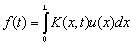0 t L,               (9)

where it will be assumed that the function f is even and periodic

f(x) = f(x), L x L.                     (10)

This condition enables f to be expanded into a Fourier cosine series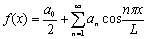(11)

where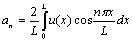(12)

Equation (10) is imposed merely as a matter of convenience. Without it, equation (11) would be changed so that u(x) is expanded into its full Fourier series . We make the obvious assumption that (11) converges almost everywhere to u(x). In order to evaluate the Fourier coefficients (12), the function must be integrable. This condition can be relaxed somewhat and other Euler equations have been defined for more general classes of functions.

In what follows we will refer to the Fourier cosine series (11) as the Fourier series. Substituting equation (11) into equation (9) gives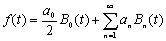(13)

where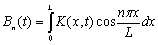(14)

According to the well known Riemann-Lebesgue theorem, if K(x, t) and u are absolutely integrable,

Then,

Bn(t) 0 and an 0 as n→∞.                (15)

Thus the high frequency terms may be neglected from (11), and we can approximate f(x) by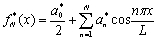(16)

for some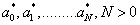. Substituting (16) into (.9), we obtain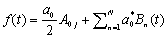(17)

To obtain the Fourier coefficients, N + 1 collocation points .

0 t0 < t1 < · · · < t N1 < tN L,           (18)

are used to produce the N + 1 equations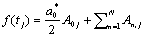, Where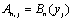j= 0, 1,..., N.                                     (19)

The corresponding matrix equation is

Aa = f,.                                                                                   (20)

where(21)

Solving (20) provides a*, a*1,..., a*N, which on substitution into (16) yields an approximation to u(x).

We mention briefly that the integrals in An,j were performed by first subdividing at the zeros of the integrand and then using a combination of Gauss-Legendre and Gauss-Log quadrature . That is(22)

The first and last integrands in (22) are not singular and the integrals are evaluated using a Gauss-Legendre rule over each zero of the integrand, the middle two integrals exhibit a logarithmic singularity and they are evaluated by a combination of Gauss-Log and Gauss-Legendre rules over the zero’s of the respective integrands. In this way, the logarithmic singularity and the high frequency nature of the integrands are both accounted for.

3. Results

The Fourier series approximation performs reasonably for small N. The exact solution with the approximation are coincide for N = 2. The error is around 2%, which is quite acceptable for this low order expansion. As expected most of the error occurs at the boundary points x = 0 and x = 1 [5, 6, 7]. This is typical of spectral expansions in that the endpoints have the slowest convergence rate and is due to the fact that at these points the coefficients, a*n, are summed with the greatest magnitude.

It is shown in the following table, the relative error in each Fourier coefficient for N = 4, 8 and 16. The error increases with n except for the last coefficient. The breakdown in the last few terms is quite large where the relative error is 28% for N = 8 and 53% for N = 16 The large increase in errors near n = N for N = 64 and N = 128 prevent the series from realizing its previous convergence rate.

As N doubles from N = 4, to N = 32, the error decreases by an order of magnitude.

The error then begins to increase, and for N = 64 and 128.

The error is largest at x = 1.

Table 1. Error in the coefficients of the Fourier series for N = 4, N = 8 and N = 16.

 n an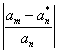N=4 N= 8 N=16 0 1.0666666666666667 0.2396E-04 0.1126E-05 0.5179E-07 1 0.4927671482248482 0.7770E-04 0.2725E-05 0.1090E-06 2 -0.0307979467640530 0.4306E-02 0.8200E-04 0.3437E-05 3 -0.0019248716727533 0.7569E-01 0.6883E-03 0.1582E-04 4 -0.0019248716727533 0.1443E-0.2 0.4154E-0.2 0.8204E-04 5 -0.0007884274371598 0.1882E-01 0.2402E-03 6 -0.0003802215649883 0.7640E-01 0.6903E-03 7 -0.0002052341308725 0.2796E+00 0.1753E-02 8 -0.0000751054943187 0.9088E-02 9 0.0000751054943187 0.9088E-02 10 0.0000492767148225 0.1846E-01 11 -0.0000336566592599 0.3886E-01 12 -0.0000237638478118 0.7467E-01 13 -0.0000172531475867 0.1482E+00 14 -0.0000128271331795 0.2771E+00 15 -0.0000097336720637 0.5308E+00 16 -0.0000075190299717 0.7605E-02

4. Conclusion

The Fourier series method successfully solved the integral equation, but the results were disappointing.

The error deteriorated after an expansion of 32 terms and this is due to the great difficulty of finding high frequency contributions to the unknown in first kind equations. Measures taken to address this point were somewhat successful and a more detailed investigation of the expansion method is warranted. This is especially true if one notes that the traction of an axisymmetric particle is inherently periodic.:

The approximation is valid for the entire domain as no discretization is employed.

The properties of the solution are derived from those of the basis functions { ϕn }. For orthogonal polynomials, and in particular trigonometric polynomials, this would mean that the solution is everywhere analytic. This may not be expedient if one knows that the solution should exhibit some non-analytic behavior. Usually, this property is desirable and non-analytic functions can be catered for by expanding in a weighted series.

References

1. Abbott, P. "Tricks of the Trade: Legendre-Gauss Quadrature." Mathematica J. 9, 689-691, 2005.
2. Abramowitz, M. and I. A. Stegun (1965). Handbook of Mathematical Functions. NewYork: Dover.
3. Atkinson, K. E. (1989). An Introduction to Numerical Analysis. New York: John Wiley andSons.
4. Bengt Fornberg (1996) A Practical Guide to Pseudospectral Methods. Cambridge University Press, Cambridge, UK
5. C. A. Brebbia, New Developments in Boundary Element Methods, Butterworths, 1980.
6. C. A. Brebbia, Boundary Element Techniques in Computer-Aided Engineering, NATO ASI Series, 1984.
7. C. A. Brebbia, Topics in Boundary Element Research, Vol. 1, Springer-Verlag, 1984.
8. Canuto C., Hussaini M. Y., Quarteroni A., and Zang T.A. (2006) Spectral Methods. Fundamentals in Single Domains. Springer-Verlag, Berlin Heidelberg
9. D. M. Hough and J. Levesley and S. N. Chandler-White, Numerical conformal mapping via Chebyshev weighted solutions of Symm's integral equation, Journal of Computational and Applied Mathematics, Vol. 46 (1993), 29-48.
10. E. S. Shoukralla, S. A. EL-Serafi and Nermein. Saber,"A Modified Iterative Method for the Solution of Fredholm Integral Equation of the Second Kind Via Matrices" International Journal of Universal Mathematics and Mathematical Sciences, 01 Issues No.: 02, ISSN No.: 2454-7271, Dec 2015.
11. G. C. Hsiao, W. L. Wendland, Boundary Integral Equations, Springer, 2008.
12. G. H. Hardy and J. E. Littlewood, Some new properties of Fourier constants, in Collected Papers of G. H. Hardy III, Oxford University Press (1969), 348-399.
13. G. Fairweather and R. L. Johnston, The method of fundamental solutions for problems in potential theory in Treatment of Integral Equations by Numerical Methods (Ed. by C. T. H. Baker and G. F. Miller), Academic Press Inc. (London) Ltd.
14. J. CF. Telles and L. C. Wrobel, Boundary Element Techniques, Springer-Verlag, 1984.
15. Jafarian A, Esmailzadeh Z, Khoshbakhti L. A numerical method for solving nonlinear integral equations in the Urysohn form. Applied Mathematical Sciences. 2013; 7(28): 1375–1385.
16. John P. Boyd,Chebyshev and Fourier Spectral Methods, DOVER Publications (2000), Inc. 31 East 2nd Street Mineola, New York 11501.
17. M. JLELI, M. MURSALEEN, B. SAMET, Q-Integral Equations of Fractional Orders, Electronic Journal of Differential Equations, Vol. 2016 (2016), No. 17, pp. 1–14. ISSN: 1072-6691. URL: http://ejde.math.txstate.edu or http://ejde.math.unt.edu ftp ejde.math.txstate.edu
18. M. A. Jaswon and G. T. Symm, Integral Equation Methods in Potential Theory and Elastostatics, Academic Press, London 1977.
19. Maleknejad K, Derili H. The collocation method for Hammerstein equations by Daubechies wavelets. Applied Mathematics and Computation. 2006;172(2): 846–864.
20. Shoukralla, E. S., EL-Serafi, S. A., Saber, Nermein A, "A matrix Iterative technique for the solution of Fredholm Integral Equations of the second kind" "Electronic Journal of Mathematical Analysis and Applications" Vol. 4(1) Jan. 2016, pp. 192-196.ISSN: 2090-729.
21. Van Tuyl, Andrew H. (1994). "Acceleration of convergence of a family of logarithmically convergent sequences" (PDF). Mathematics of Computation. 63 (2007): 229–246. doi:10.2307/2153571.

 Contents 1. 2. 3. 4.
Article ToolsAbstractPDF(187K)